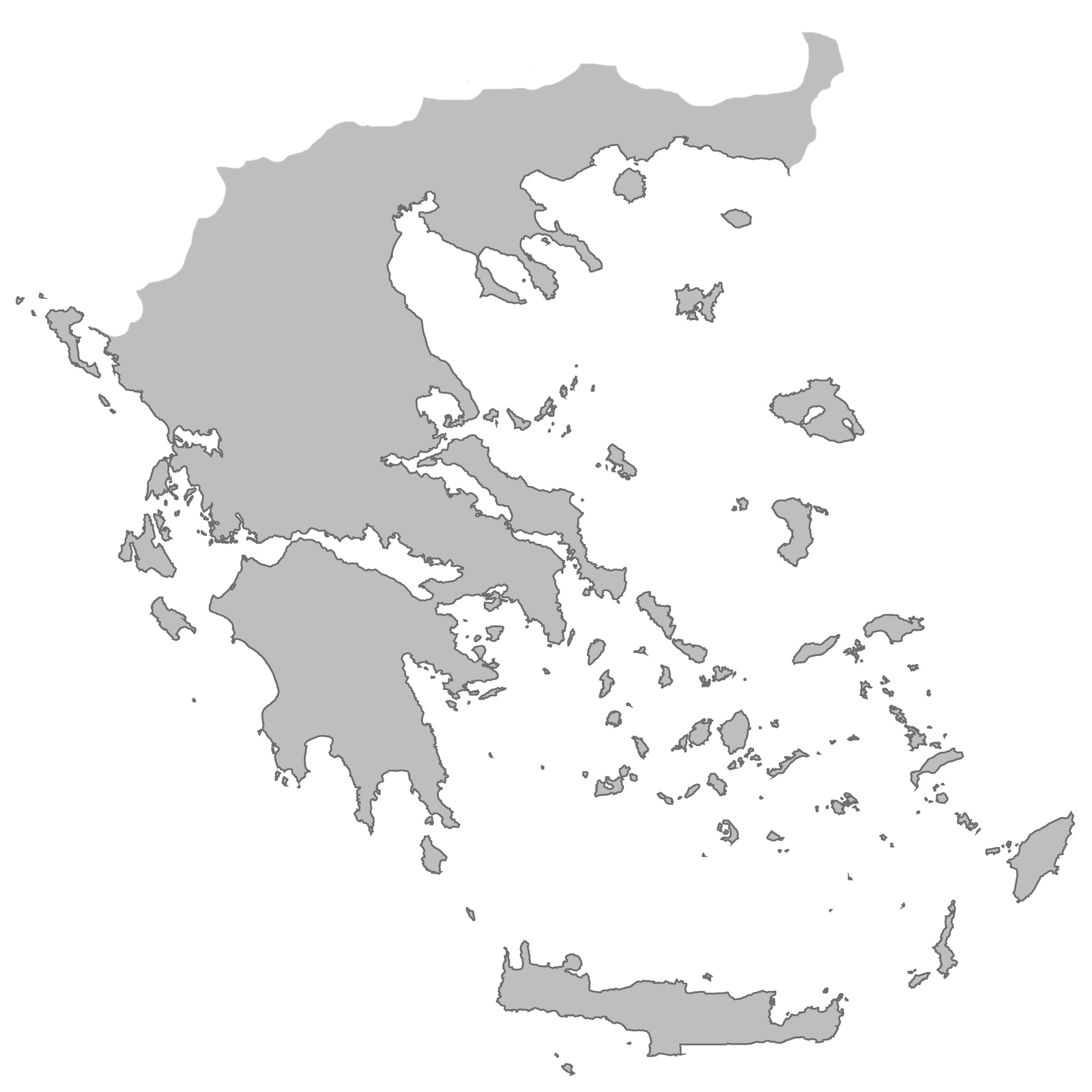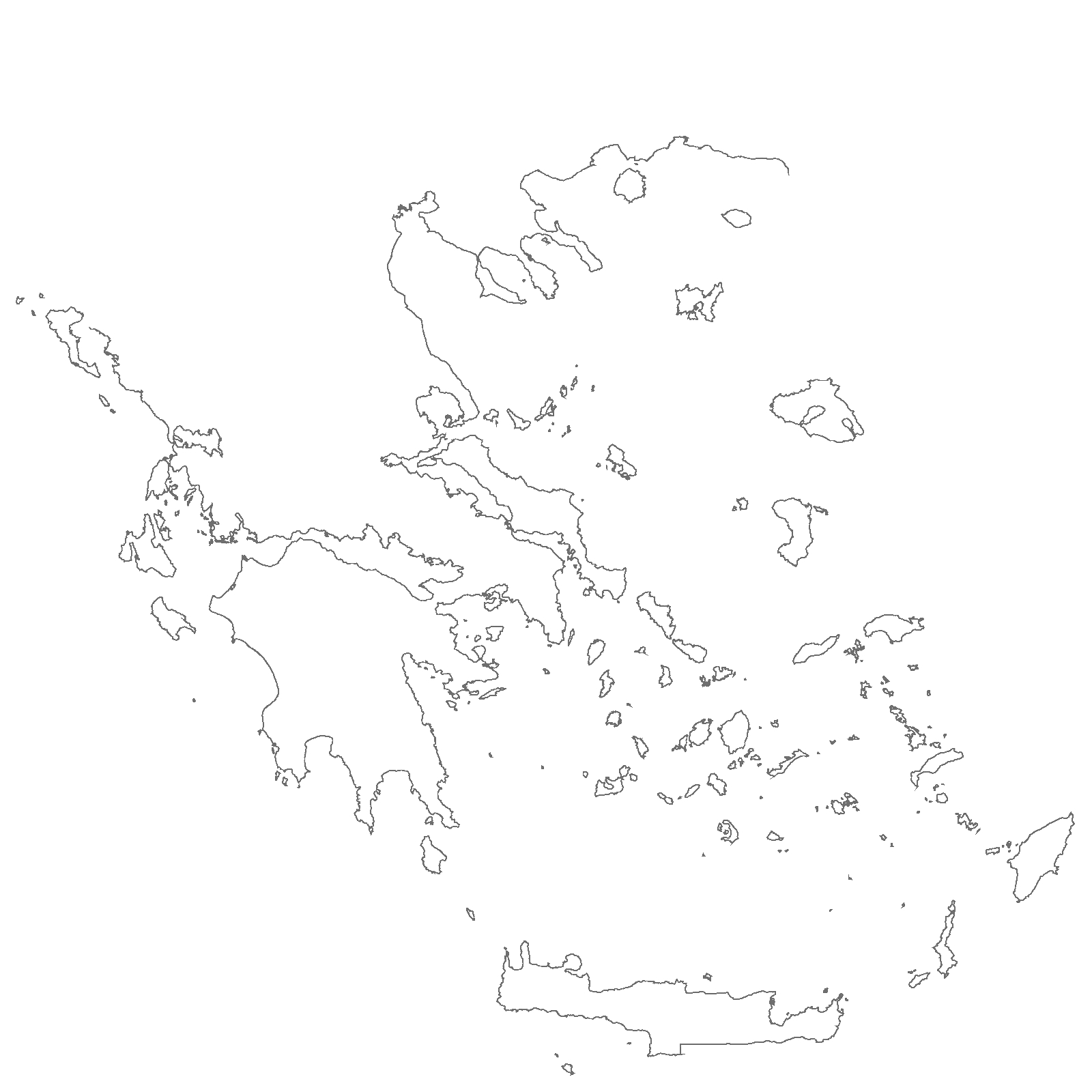Calculating the fractal dimension of the Greek coastline (1.25)

by Kyriakos Chatzidimitriou | Apr 19, 2013 00:54 | tutorials

complexityfractal dimensionfractalsgreek coastliner

Inspired by the Introduction to Complexity course and the unit on Factals, I though it would be fun to make a rough calculation of the fractal dimension of the Greek coastline using the box counting method.

The box counting method goes as follows:

1. Split the 2D map that depicts the coastline into squares (boxes) of a certain side size (r) and count the number of boxes (n) that include the coastline.
2. Decrease the size of the boxes and go to step 1.
3. When finished for a series of box sizes do a linear regression of log(n) given log(1/r).
4. The slope of the line fitting the points on the plot is the fractal dimension of the object.
For a map of Greece, I used the one from Ginko maps licensed under the Creative Commons Attribution 3.0. Via an image editor, I removed the frame with the infobox and geolocation axes, plus the borders not residing by the sea to facilitate further image processing. The retouched image was cropped to 1600×1600 pixels. Both images are shown below.

## Original map## Retouched mapThe R script below implements the box counting method on the coastline jpeg picture (make all values > 0.5 white) using boxes with sides that are divisors of 1600.

## The coastline map## The R script


library(jpeg)
rm(list=ls())
# filter out mainland
img[img > 0.5] = 1
# divisors of 1600
# 1,2,4,5,8,10,16,20,25,32,40,50,64,80,100,160,200,320,400,800,1600
boxSizes = c(50, 40, 32, 25, 20, 16, 10, 8, 5, 4)
h = img[,,1]
data = data.frame()
for(k in 1:length(boxSizes)) {
b = boxSizes[k]
x = dim(img[,,1])
ratio = x/b
# https://stat.ethz.ch/pipermail/r-help/2012-February/303163.html
k = kronecker(matrix(1:(ratio^2), ratio, byrow = TRUE), matrix(1,b,b))
g = lapply(split(h,k), matrix, nr = b)
counter = 0;
for(i in 1:length(g)) {
counter = counter + any(g[[i]] < 0.999)
}
data = rbind(data,c(log(counter),log(1/b)))
}
names(data) = c("Y", "X")
model = lm(Y~., data=data)
cat(coef(model), "\n")


## The plotWith this rough approximation, the calculation yielded that the fractal dimension of the Greek coastline is 1.25. Great Britain’s was measured to be 1.25 and Norway’s 1.52 [source].